CBSE Class 12 Sample Paper for 2019 Boards

Class 12
Solutions of Sample Papers and Past Year Papers - for Class 12 Boards

## Find the equations of the normal to the curve y = 4x 3 – 3x + 5 which are perpendicular to the line 9x – y + 5 = 0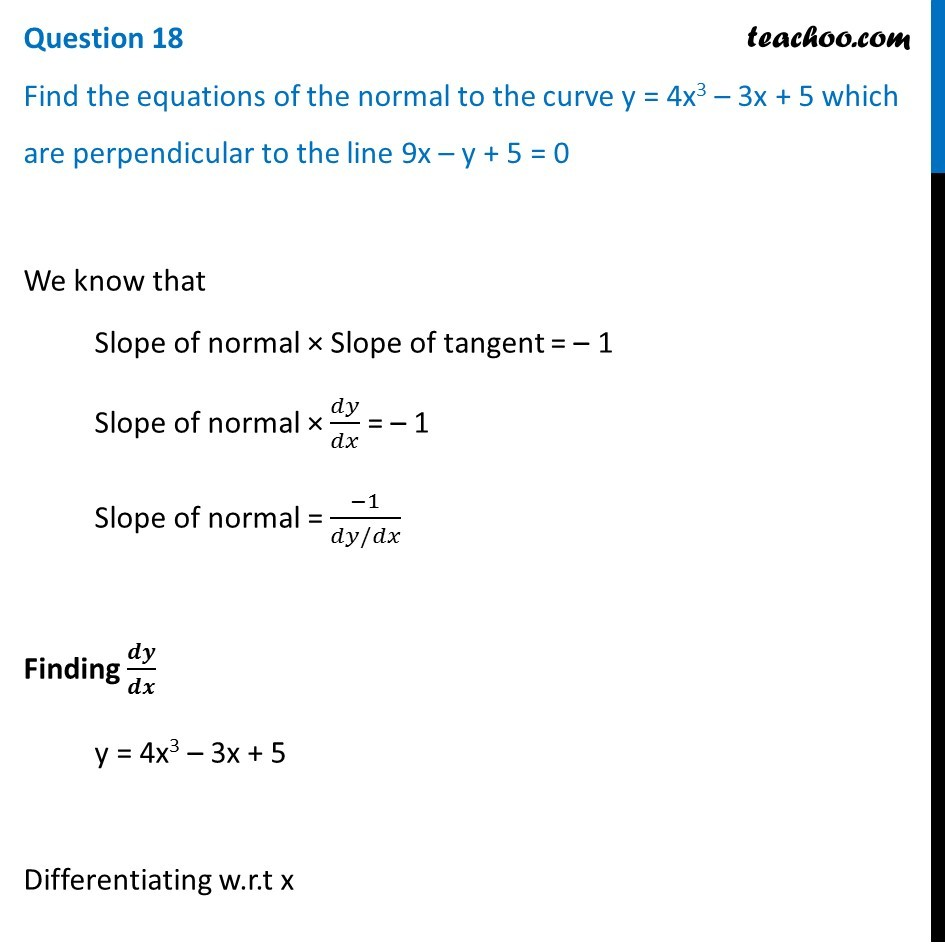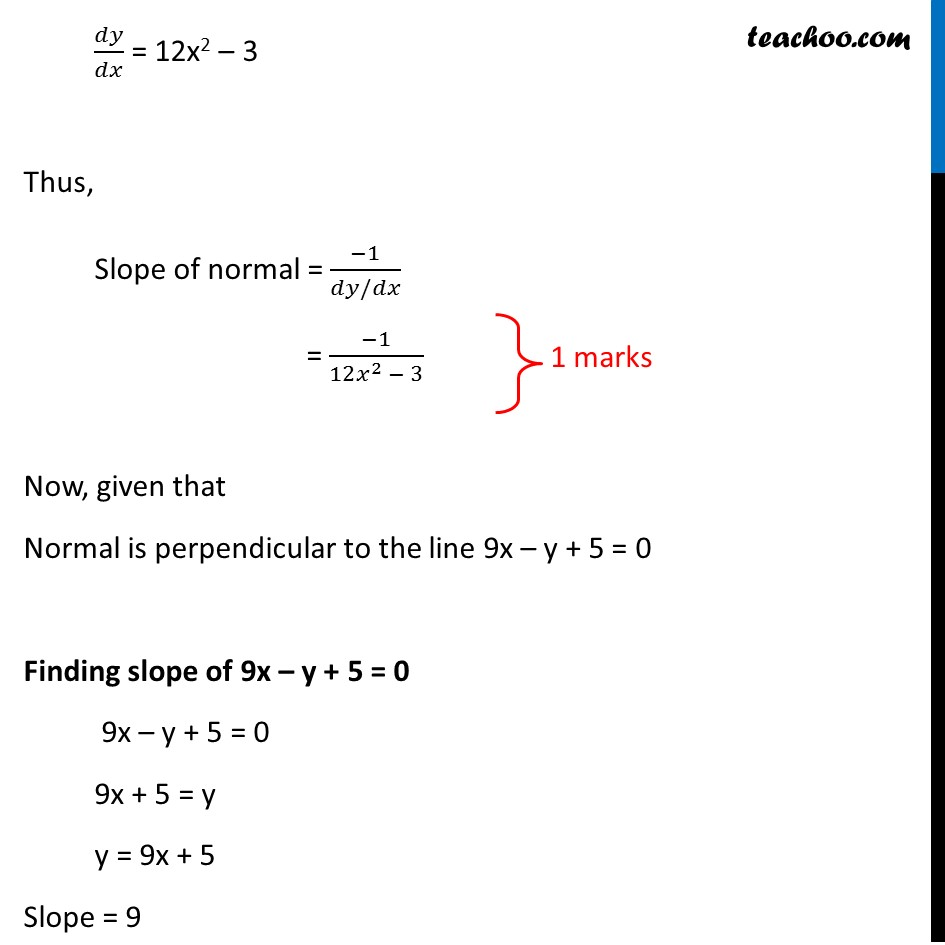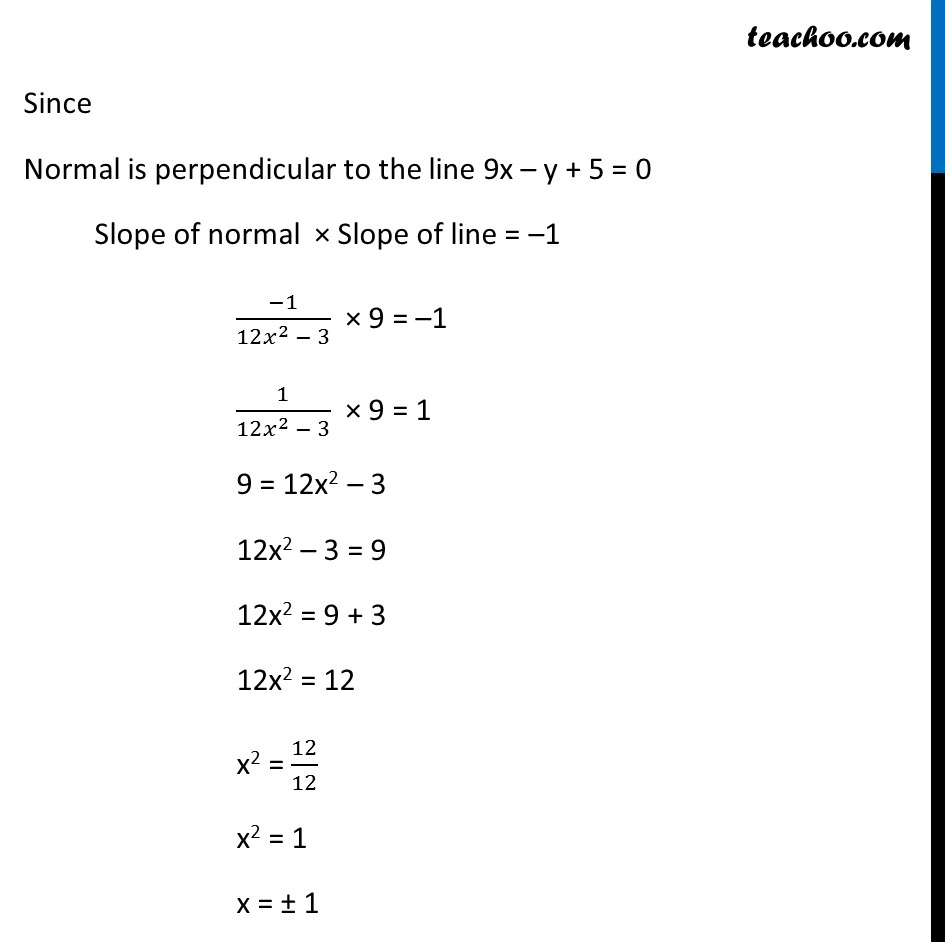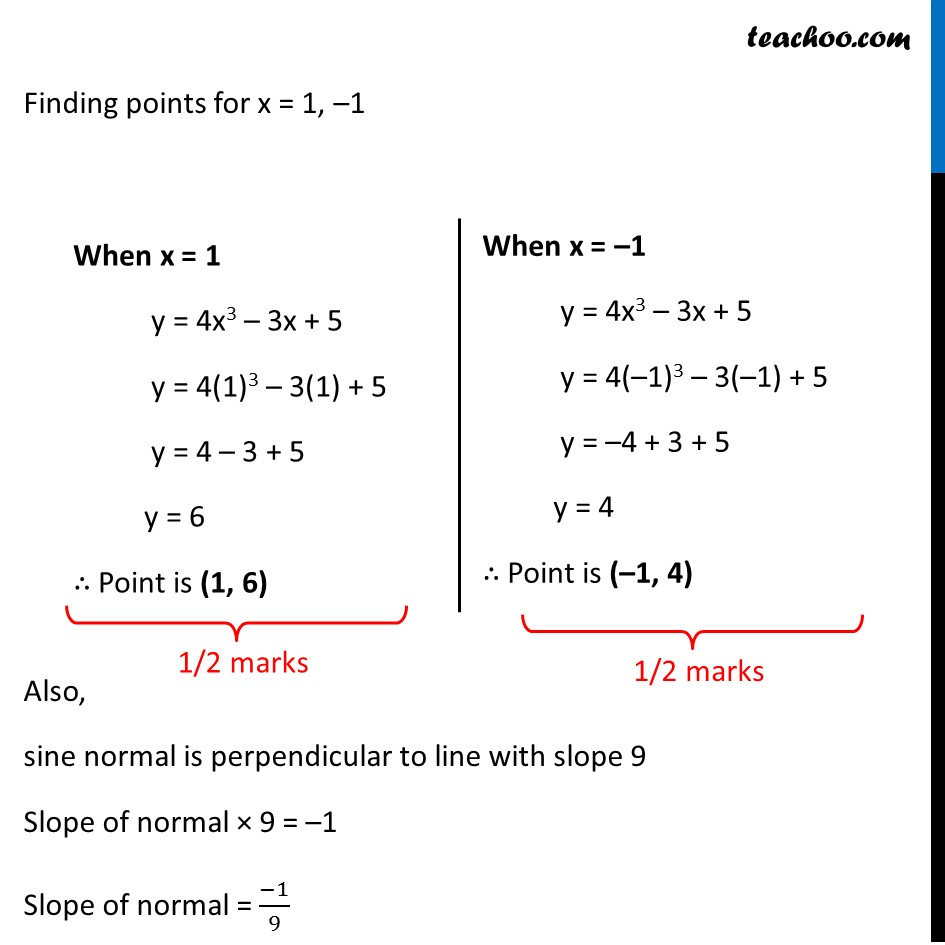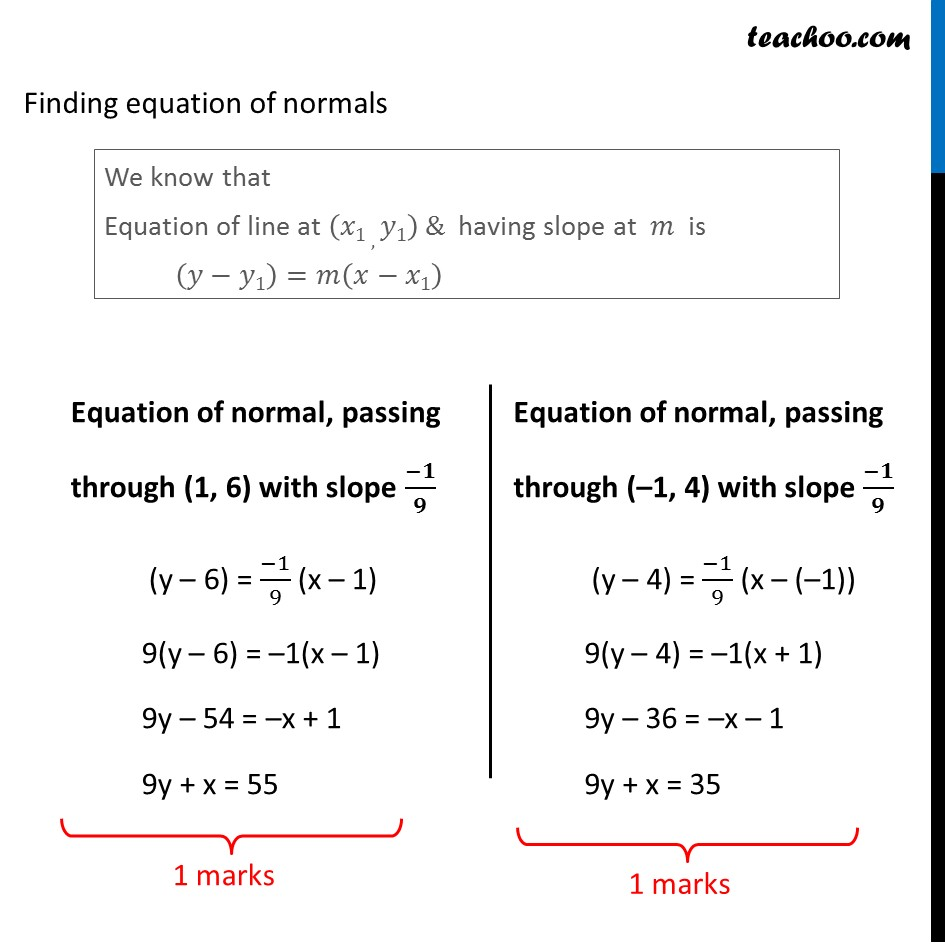Learn in your speed, with individual attention - Teachoo Maths 1-on-1 Class

### Transcript

Question 18 Find the equations of the normal to the curve y = 4x3 – 3x + 5 which are perpendicular to the line 9x – y + 5 = 0 We know that Slope of normal × Slope of tangent = – 1 Slope of normal × 𝑑𝑦/𝑑𝑥 = – 1 Slope of normal = (−1)/(𝑑𝑦/𝑑𝑥) Finding 𝒅𝒚/𝒅𝒙 y = 4x3 – 3x + 5 Differentiating w.r.t x 𝑑𝑦/𝑑𝑥 = 12x2 – 3 Thus, Slope of normal = (−1)/(𝑑𝑦/𝑑𝑥) = (−1)/(12𝑥^2 − 3) Now, given that Normal is perpendicular to the line 9x – y + 5 = 0 Finding slope of 9x – y + 5 = 0 9x – y + 5 = 0 9x + 5 = y y = 9x + 5 Slope = 9 Since Normal is perpendicular to the line 9x – y + 5 = 0 Slope of normal × Slope of line = –1 (−1)/(12𝑥^2 − 3) × 9 = –1 1/(12𝑥^2 − 3) × 9 = 1 9 = 12x2 – 3 12x2 – 3 = 9 12x2 = 9 + 3 12x2 = 12 x2 = 12/12 x2 = 1 x = ± 1 Finding points for x = 1, –1 When x = 1 y = 4x3 – 3x + 5 y = 4(1)3 – 3(1) + 5 y = 4 – 3 + 5 y = 6 ∴ Point is (1, 6) When x = –1 y = 4x3 – 3x + 5 y = 4(–1)3 – 3(–1) + 5 y = –4 + 3 + 5 y = 4 ∴ Point is (–1, 4) Also, sine normal is perpendicular to line with slope 9 Slope of normal × 9 = –1 Slope of normal = (−1)/9 Finding equation of normals We know that Equation of line at (𝑥1 ,𝑦1) & having slope at 𝑚 is (𝑦−𝑦1)=𝑚(𝑥−𝑥1) Equation of normal, passing through (1, 6) with slope (−𝟏)/𝟗 (y – 6) = (−1)/9 (x – 1) 9(y – 6) = –1(x – 1) 9y – 54 = –x + 1 9y + x = 55 Equation of normal, passing through (–1, 4) with slope (−𝟏)/𝟗 (y – 4) = (−1)/9 (x – (–1)) 9(y – 4) = –1(x + 1) 9y – 36 = –x – 1 9y + x = 35